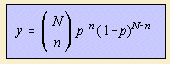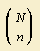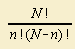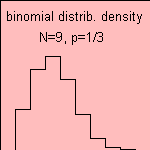# binomial distribution density

## discrete curve

last updated: 2004-05-01whereis called the binomial coefficient ' N over n', what stands for the number of combinations when taking n objects out a collection of N objects.
The formula is an abbreviation of:Suppose that an event has a probability p to occur. Examples of such events are  'heads or tails', and the chance on a certain number on a dice. The binomialdistribution gives the probability of n successes for a number of N events.

When p=1/2, we find the binomial: